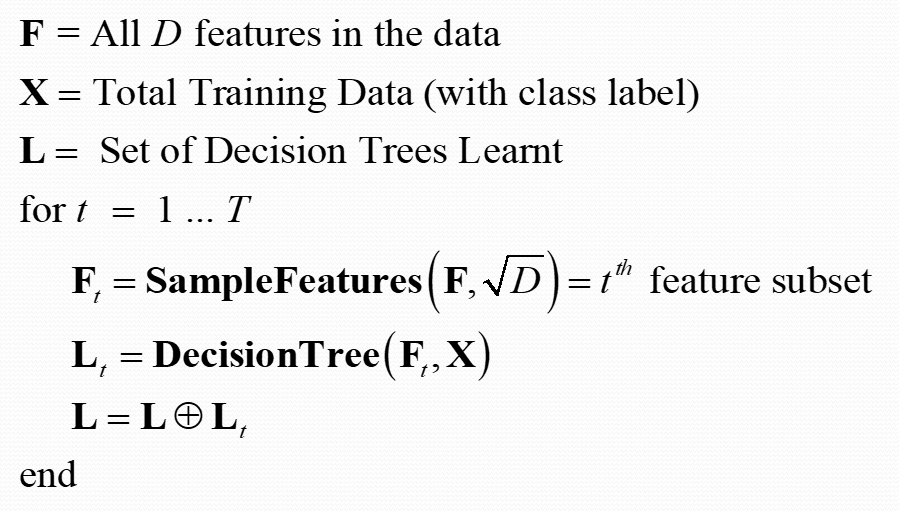### Basic Introduction to Random Forest

Decision Tree is one of the most used classical machine learning algorithms. But One of the biggest drawbacks of Decision Tree is that they are highly prone to overfitting. So to minimize this problem, we use Random Forest. In this article, we will study the basics of Random Forest and various terminologies related to it.

1. Random Forest is used for an ensemble of decision trees. It uses the base principle of bagging with random feature selection to create more diverse trees.
2. Splitting a node during the construction of a tree, the split that is chosen is no longer the best split among all the features. Instead, the split picked is the best split among a random subset of the features
3. As a result of this randomness, the bias of the forest usually slightly increases (with respect to the bias of a single non-random tree)
4. Due to averaging, its variance decreases, usually more than compensating the increase in bias, hence yielding overall a better result.
5. Can handle curse of dimensionality as the ensemble uses only a small random portion of the full feature set. Less prone to over-fitting.
6. Select Sqrt(P) where P = number of features

### TRAINING PHASEAlgorithm to Train Random Forest

### Lets explore Hyperparameters in Random Forest:

Random Forest has a lot of parameters, because of which cross-validation phase to find an optimal set of parameters takes a very long time.

1. n_estimators: integer, optional (default=10)The number of trees in the forest.
2. criterion: string, optional (default=”gini”)The function to measure the quality of a split. Supported criteria are “gini” for the Gini impurity and “entropy” for the information gain.
3. max_depth: integer or None, optional (default=None)The maximum depth of the tree. If None, then nodes are expanded until all leaves are pure or until all leaves contain less than min_samples_split samples.
4. max_features: int, float, string or None, optional (default=”auto”)The number of features to consider when looking for the best split:
5. bootstrap : boolean, optional (default=True)
6. Whether bootstrap samples are used when building trees. If False, the whole dataset is used to build each tree.
7. oob_score: bool (default=False) Whether to use out-of-bag samples to estimate the generalization accuracy.
8. warm_start: bool, optional (default=False)When set to True, reuse the solution of the previous call to fit and add more estimators to the ensemble, otherwise, just fit a whole new forest.
9. n_jobs: int or None, optional (default=None) The number of jobs to run in parallel for both fit and predict. None means 1 and -1 means using all processors.

### Advantages of a Random Forest

1. A random forest is more stable than any single decision tree because the results get averaged out; it is not affected by the instability and bias of an individual tree.
2. A random forest is immune to the curse of dimensionality since only a subset of features is used to split a node.
3. You can parallelize the training of forest since each tree is constructed independently.

4. You can calculate the OOB (Out-of-Bag) error using the training set which gives a really good estimate of the performance of the forest on unseen data.
Hence there is no need to split the data into training and validation; you can use all the data to train the forest.

### The OOB error:

The OOB error is calculated by using each observation of the training set as a test observation. Since each tree is built on a bootstrap sample, each observation can be used as a test observation by those trees which did not have it in their bootstrap sample.

All these trees predict this observation and you get an error for a single observation. The final OOB error is calculated by calculating the error on each observation and aggregating it. It turns out that the OOB error is as good as a cross-validation error.

You May Like: